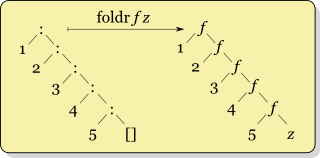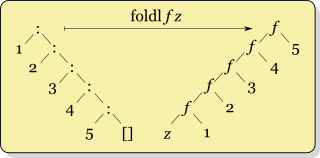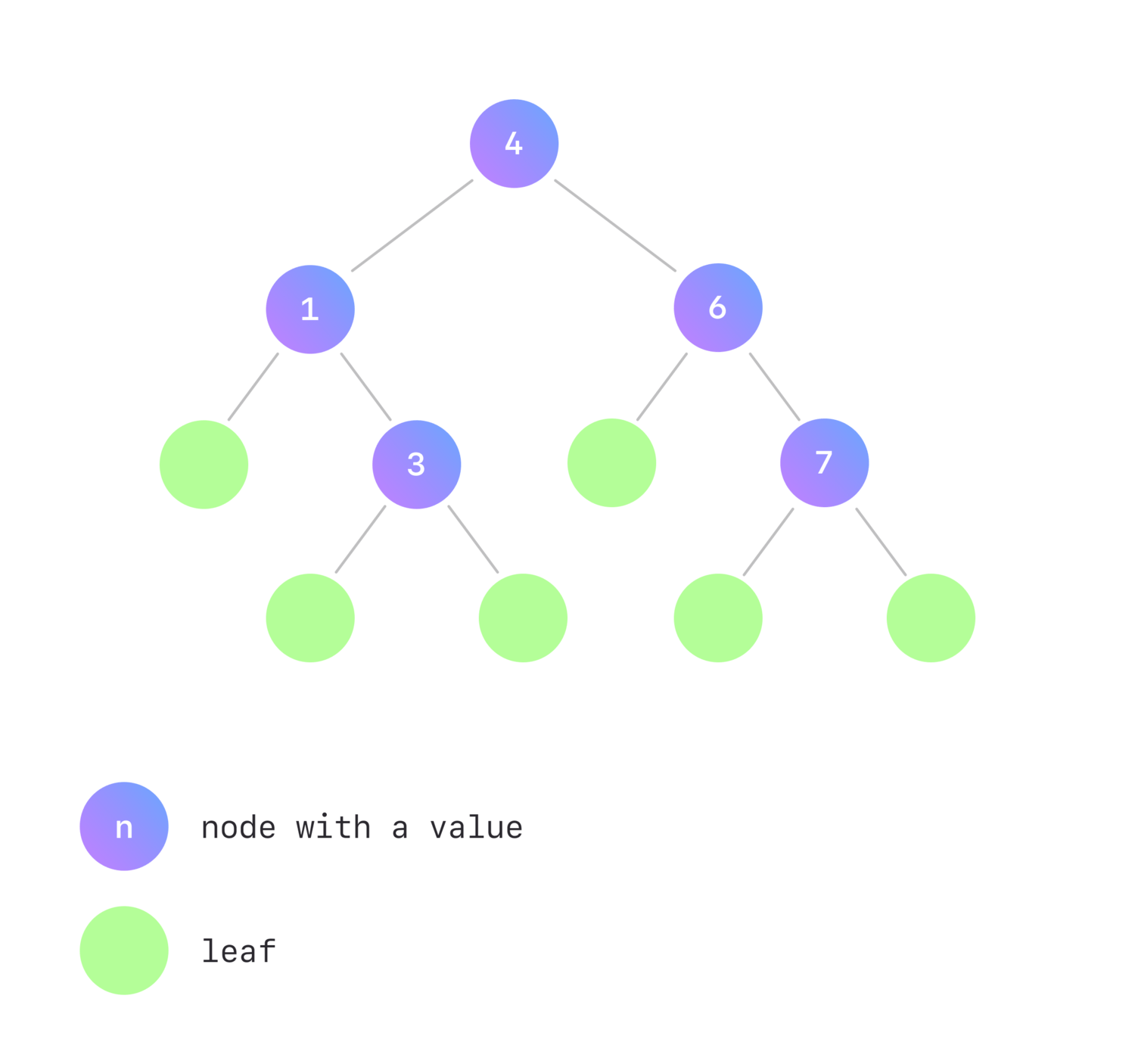# What's That Typeclass: Foldable

There is a function called reduce in programming languages, which can be applied to some containers. It goes element by element, applying a given binary function and keeping the current result as an accumulator, finally returning the value of the accumulator as a summary value of the container.

For example, in Python:

> reduce(lambda acc, elem: acc + elem, [1, 2, 3, 4, 5]) # sum of elements in a list
15


Haskell, in turn, has two fundamental functions representing reducing, or, as we call it, folding – foldl and foldr – that differ in the order of the folding. foldl reduces elements of a container from left to right (as reduce in other languages usually does), while foldr reduces from right to left.

These two functions are core methods of the Foldable type class, about which we are going to learn in this article.

## foldr and foldl for a list

First, let’s figure out what foldr and foldl are for a list.

Consider the following type signatures:

foldr :: (a -> b -> b) -> b -> [a] -> b
foldl :: (b -> a -> b) -> b -> [a] -> b


### foldr

The foldr signature corresponds to the function folding the list from right to left. It takes a function f of type a -> b -> b as the first argument, an initial (or base) value z of type b as the second argument, and a list xs = [x₁, x₂, ..., xₙ] of type [a] as the third one.

Let’s trace it step-by-step.

1. Introduce an accumulator acc, which is equal to z at start.
2. f is applied to the last element of the list (xₙ) and a base element z, the result of which is written in the accumulator – xₙ f z.
3. f is applied to the second-to-last element of the list and the current value of the accumulator – xₙ₋₁ f (xₙ f z).
4. After going through all the elements of the list, foldr returns the accumulator as a summary value, which finally equals to x₁ f (x₂ f ... (xₙ₋₁ f (xₙ f z)) ... ).
acc = z
acc = xₙ f z
acc = xₙ₋₁ f (xₙ f z)
...
acc = x₁ f (x₂ f ( ... (xₙ₋₁ f (xₙ f z)) ... )


This process could be represented as a picture:### foldl

Unlike foldr, foldl performs left-to-right folding of a list. Therefore, f is applied to z and x₁ first – acc = z f x1 – then f is applied to the current value of the accumulator and x₂acc = (z f x₁) f x₂ – and so on. Finally, foldl returns acc = ( ... ((z f x₁) f x₂) f ... xₙ₋₁) f xₙ.

acc = z
acc = z f x₁
acc = (z f x₁) f x₂
...
acc = ( ... (z f x₁) f x₂) f ...) f xₙ₋₁) f xₙ


The corresponding illustration of these operations:The recursive Haskell definition of foldr is the following:

instance Foldable [] where
foldr :: (a -> b -> b) -> b -> [a] -> b
foldr _ z []     =  z
foldr f z (x:xs) =  x f foldr f z xs


foldl is similar; implementing it is one of the exercises below.

### Simple examples

Let’s consider a couple of simple examples of applying foldr and foldl to a list:

-- addition
ghci> foldr (+) 0 [1, 2, 3] -- 1 + (2 + (3 + 0))
6
ghci> foldl (+) 0 [1, 2, 3] -- ((0 + 1) + 2) + 3
6

-- exponentiation
ghci> foldr (^) 2 [2, 3] -- 2 ^ (3 ^ 2)
512
ghci> foldl (^) 2 [2, 3] -- (2 ^ 2) ^ 3
64


As you can see, it doesn’t matter to the addition if the folding is right-to-left or left-to-right since its operator is associative. However, when folding with an exponentiation operator, the order is significant.

## Generalization of foldr and foldl

Fortunately, it’s possible to fold not only lists! We can implement the instance of Foldable whenever a data type has one type argument, in other words, when its kind is * -> *.

To figure out the kind of Haskell data type, you can write :kind (or :k) in GHCi. To display the type of a Haskell expression, use :type (or :t).

For instance, a list has one type argument – the type of the list’s elements:

ghci> :kind []
[] :: * -> *

ghci> :type [1 :: Int, 2, 3]
[1, 2, 3] :: [Int]
ghci> :type ['a', 'b']
['a', 'b'] :: [Char]


Maybe a data type also has one type argument – the type of presented value:

ghci> :kind Maybe
Maybe :: * -> *

ghci> :type Just (2 :: Int)
Just 2 :: Maybe Int
ghci> :type Just "abc"
Just "abc" :: Maybe [Char]
ghci> :type Nothing
Nothing :: Maybe a -- can't decide what type a is


Int and String have no type arguments, and you can’t fold them:

ghci> :kind Int
Int :: *
ghci> :type (1 :: Int)
1 :: Int

ghci> :kind String
String :: *
ghci> :type ""
"" :: [Char]
ghci> :type "ab"
"ab" :: [Char]


Either a b data type has two type arguments corresponding to Left and Right values, so there is also no reasonable way to fold an Either a b. But we could define instance Foldable (Either a) since Either a has an appropriate * -> * kind. The same for the pair data type – instance Foldable ((,) a) – is possible. However, such instances are not intuitive since they operate on only one of type arguments.

ghci> :kind Either
Either :: * -> * -> *

ghci> :type Left (1 :: Int)
Left 1 :: Either Int b -- can't decide what type argument b is
ghci> :type Right 'a'
Right 'a' :: Either a Char -- can't decide what type argument a is

ghci> :kind Either Int
Either Int :: * -> *
ghci> :kind (,) Char
(,) Char :: * -> *


For those data types which could have a Foldable instance, generalized versions of foldr and foldl have the following type signatures:

foldr :: Foldable t => (a -> b -> b) -> b -> t a -> b
foldl :: Foldable t => (b -> a -> b) -> b -> t a -> b


### Some instances as an example

#### Maybe a

Let’s take a look at other instances of Foldable. One easy-to-understand example is Maybe a’s instance. Folding Nothing returns a base element, and reducing Just x with foldr f z (or foldl f z) is applying f to x and z:

instance Foldable Maybe where
foldr :: (a -> b -> b) -> b -> Maybe a -> b
foldr _ z Nothing  = z
foldr f z (Just x) = f x z


Usage examples:

ghci> foldr (+) 1 (Just 2)
3
ghci> foldr (+) 1 Nothing
1


#### BinarySearchTree a

A more interesting and useable Foldable instance could be defined for a BinarySearchTree a data type.

Consider a binary search tree, each node of which either:

• stores a value of type a and has left and right subtrees;
• is a leaf without a value.

Moreover, for each non-leaf node n:

• all values in its left subtree should be less or equal than the n’s value;
• and all values in its right subtree should be greater than the n’s value.This structure matches the following Haskell definition:

data BinarySearchTree a
= Branch (BinarySearchTree a) a (BinarySearchTree a)
| Leaf


Imagine that we want to reduce the whole tree to just one value. We could sum values of nodes, multiply them, or perform any other binary operation. So, it’s reasonable to define a folding function that matches your goals. We suggest you try to implement a Foldable instance on your own; it’s one of the exercises below. Take into account that to define foldr, we need to go through the tree’s elements from right to left – from right subtree to left subtree through the non-leaf node connecting them.

## What do you need to define a Foldable instance?

To answer this question, we need to look into another method of Foldable, foldMap.

### foldMap

foldMap has the following declaration:

foldMap :: (Monoid m, Foldable t) => (a -> m) -> t a -> m


It’s important to note that foldMap has a Monoid constraint. Its first argument is a function that maps each element of a container into a monoid, the second argument is a container itself. After mapping elements, the results are combined using (<>) operator. The order of folding is from right to left, so foldMap could be implemented via foldr.

Let’s look at how exactly that could be done:

foldMap :: (Monoid m, Foldable t) => (a -> m) -> t a -> m
foldMap f = foldr (\x acc -> f x <> acc) mempty


foldMap doesn’t have a base element since only the elements of the container are reduced. However, foldr does, so it perfectly makes sense to use the identity of monoid – mempty. We can also see that the folding function f is composed with (<>), thus, the current result is appended to a monoid accumulator on each step.

Recall how list elements can be summed with foldr:

ghci> foldr (+) 0 [1, 2, 3]
6


To do the same in terms of monoid, consider a monoid under addition – Sum. We can use foldMap and Sum to perform the addition of list elements:

-- import Data.Monoid to get Sum
ghci> import Data.Monoid

ghci> foldMap Sum [1, 2, 3]
Sum {getSum = 6}


Note that here constructor Sum is a function that turns a list element into monoid. As expected, we get the same result wrapped in Sum that can easily be accessed via getSum.

To conclude, foldMap and foldr are interchangeable, the difference is that the former receives the combiner and the base element from the Monoid instance, and the latter accepts them explicitly.

### Minimal complete definition

We have already shown how foldMap is implemented using foldr. It’s not obvious, but foldr could also be implemented via foldMap! It’s off-topic for this article, therefore, please proceed to the documentation for the details.

Consequently, to create the Foldable instance, you can provide a definition of either foldr or foldMap, which is exactly the minimal complete definition – foldMap | foldr. Note that you shouldn’t implement foldMap in terms of foldr and simultaneously foldr in terms of foldMap since this will just loop forever. Thus, you implement one of them, and Haskell provides the definitions of all Foldable’s methods automatically.

## Strict foldl'

Haskell uses lazy evaluation by default, and it can have a positive effect on performance in a lot of cases. But laziness might also impact it negatively sometimes, and folding is exactly this case.

Imagine that you want to sum up the elements of a really huge list. When using foldl or foldr, the value of the accumulator isn’t evaluated on each step, so a thunk is accumulated. Considering foldl, in the first step the thunk is just z f x₁, in the second – (z f x₁) f x₂, that’s not a big deal. But in the final step, the accumulator stores ( ... (z f x₁) f x₂) f ...) f xₙ₋₁) f xₙ. If the list’s length is above ~10^8, the thunk becomes too large to store it in a memory, and we get ** Exception: stack overflow. However, that’s not the reason to drop Haskell since we have foldl'!

foldl' enforces weak head normal form in each step, thus preventing a thunk from being accumulated. So foldl' is often a desirable way to reduce a container, especially when it’s large, and you want a final strict result.

Here are some “benchmarks” that may vary depending on a computer’s characteristics. But they are illustrative enough to grasp the difference between the performance of a strict and a lazy fold.

-- set GHCi option to show time and memory consuming
ghci> :set +s

-- lazy foldl and foldr
ghci> foldl (+) 0 [1..10^7]
50000005000000
(1.97 secs, 1,612,359,432 bytes)

ghci> foldr (+) 0 [1..10^7]
50000005000000
(1.50 secs, 1,615,360,800 bytes)

-- lazy foldl and foldr
ghci> foldl (+) 0 [1..10^8]
*** Exception: stack overflow

ghci> foldr (+) 0 [1..10^8]
*** Exception: stack overflow

-- strict foldl'
ghci> foldl' (+) 0 [1..10^8]
5000000050000000
(1.43 secs, 8,800,060,520 bytes)

ghci> foldl' (+) 0 [1..10^9]
500000000500000000
(15.28 secs, 88,000,061,896 bytes)

ghci> foldl' (+) 0 [1..10^10]
50000000005000000000
(263.51 secs, 1,139,609,364,240 bytes)


## Other methods of Foldable

foldl, foldr, and foldMap could be considered the core for understanding the nature of Foldable. Nonetheless, there are others which are worth mentioning. You get these automatically by defining foldr or foldMap.

• length, which you might know, is implemented via foldl'.
• maximum and minimum use strict foldMap' in their definitions.
• null, which checks if a container is empty, is also implemented by reducing the latter with foldr.
• You may wonder, where is the good old for loop? Fortunately, Haskell also provides it, which would have been impossible without Foldable.

All in all, even if you use foldr and foldl themselves very seldom, you will find other primitives of Foldable quite useful. You might proceed to the documentation to get to know them better.

## Exercises

The theoretical part of our article is over. We hope that you know what Foldable is now! We suggest you to try out your new knowledge with our mini exercises.

1. Which definition is correct?

foldr (\s rest -> rest + length s) 0 ["aaa", "bbb", "s"]
foldr (\rest s -> rest + length s) 0 ["aaa", "bbb", "s"]

Solution

The first definition is correct. Recall the type signature of the foldr’s first argument – a -> b -> b. a type here corresponds to the type of container, b type, in turn, is the type of an accumulator. In this example, the length of a current string is added to the accumulator in each step, so s matches the current element which has the type of a container – a.

2. Implement foldl recursively.

Hint: look at the foldr definition above.

Solution
foldl :: (b -> a -> b) -> b -> [a] -> b
foldl _ z []     =  z
foldl f z (x:xs) =  foldl f (z f x) xs


3. Implement foldr for the BinarySearchTree a.

Bear in mind that to allow type signature in instance definition, you need to use the InstanceSigs extension.

Expected behaviour:

-- Binary search tree from the example picture above
ghci> binarySearchTree = Branch (Branch Leaf 1 (Branch Leaf 3 Leaf)) 4 (Branch Leaf 6 (Branch Leaf 7 Leaf))

ghci> foldr (+) 0 binarySearchTree
21
ghci> foldr (-) 0 binarySearchTree
3
ghci> foldl (-) 0 binarySearchTree
-21

Solution
instance Foldable BinarySearchTree where
foldr :: (a -> b -> b) -> b -> BinarySearchTree a -> b
foldr _ z Leaf                     = z
foldr f z (Branch left node right) = foldr f (node f foldr f z right) left


4. Implement a reverse function for lists via foldl.

Expected behaviour:

ghci> reverse [1, 2, 3]
[3, 2, 1]

Solution
reverse :: [a] -> [a]
reverse = foldl (\acc x -> x:acc) []


5. Implement a prefixes function for lists via foldr.

Expected behaviour:

ghci> prefixes [1, 2, 3]
[, [1, 2], [1, 2, 3]]

ghci> prefixes []
[]

Solution
prefixes :: [a] -> [[a]]
prefixes = foldr (\x acc -> [x] : (map (x :) acc)) []


6. Implement the sum of the squares via both foldr and foldMap.

Expected behaviour:

ghci> sumSquares [1, 2, 3]
14

ghci> sumSquares []
0

Solution with plain foldr
sumSquares :: [Int] -> Int
sumSquares = foldr (\x acc -> x^2 + acc) 0


Solution with foldr and map
sumSquares :: [Int] -> Int
sumSquares xs = foldr (+) 0 (map (^2) xs)


Solution with foldMap and getSum
sumSquares :: [Int] -> Int
sumSquares xs = getSum (foldMap (\x -> Sum x^2) xs)